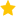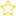Resources for teaching statistics: Key Resources, Teacher Preparation

In this video, we begin looking at scatterplots and ways of viewing and interpreting them.

After you’ve watched this video, you should be able to answer these questions:

• How do we use a scatter plot to predict a new outcome at a given value of the predictor variable?
• How can we find a range of values that is likely to contain the new outcome?
• When will the prediction intervals/ranges be wide and when will they be narrow?
• What must we assume when we use existing data to predict a new outcome?
• In what type of region is it particularly dangerous to make predictions?
• How can we check visually whether a trend line or curve is positioned properly?
• What should the trend value corresponding to a particular predictor-variable value (the value 5 was used in the video) be telling us about?

## Rate this resource:(1 ratings)